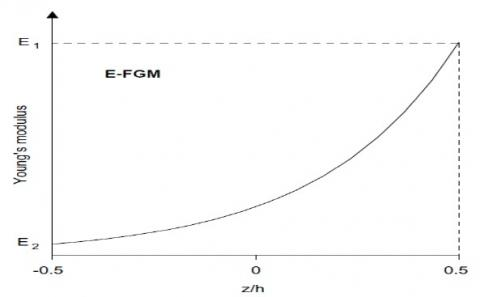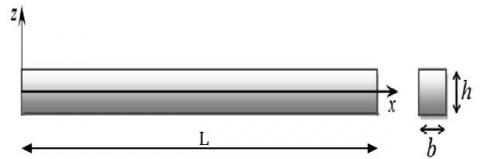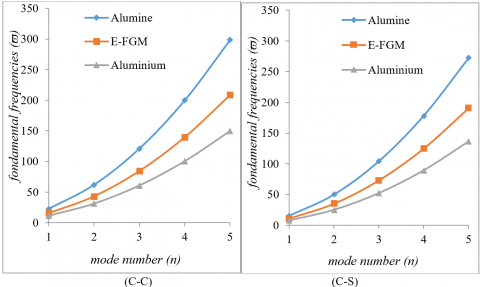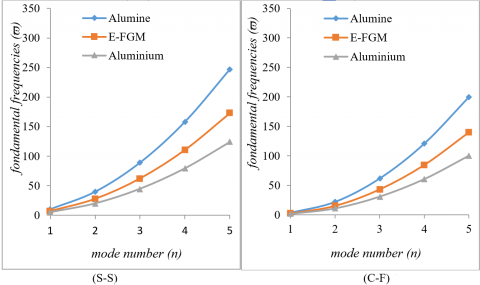Mohamed BouamamaAbbes Elmeiche Abdelhak Elhennani Tayeb Kebir Zine El Abidine Harchouche

Laboratory Mechanics of Structures and Solids, Department of Mechanical Engineering, Faculty of Technology, University of Sidi Bel Abbes, Sidi Bel Abbes 22000, Algeria

Laboratory of Materials and Systems Reactive, Department of Mechanical Engineering, Faculty of Technology, University of Sidi Bel Abbes, Sidi Bel Abbes 22000, Algeria

Corresponding Author Email:
mohamed.bouamama@univ-sba.dz
Page:
55-60
|
DOI:
https://doi.org/10.18280/rcma.300201
18 November 2019
|
Accepted:
6 January 2020
|
Published:
15 May 2020
| Citation

OPEN ACCESS

Abstract:

This study relates the exact solution for free-vibration analysis of beams in material gradient (FGMs) subjected to the different conditions of support using the Euler Bernoulli theory (CBT). It is assumed that the material properties continuously change across the thickness of the beam according to the exponential function (E-FGM). The equations of motion are obtained by applying the principle of virtual works on beams and fundamental frequencies are found by solving the equations governing the eigenvalue problems. Numerical results are presented to describe the influence of the material on the fundamental frequencies of the beam for different state boundaries.

Keywords:

exact solution, free vibration analysis, beams, E-FGM, fundamental frequencies, material distribution

1. Introduction

Improving the performance of the structural parts can lead to search, in the same material, different properties, often antagonistic, but locally optimized. The development of composite materials has made it possible to associate specific properties of different materials within the same room.

Improving the performance of the structural parts can lead to search, in the same material, different properties, often antagonistic, but locally optimized. The development of composite materials has made it possible to associate specific properties of the different materials within the same room.

Gradient property materials (FGMs) can be produced by continually changing the material components in a predetermined profile. The most distinct characteristics of the FGM micro-structure materials are their non-uniform with graded properties, macro in space. It is designed to improve and optimize the characteristic thermoelectric mechanical structures for sealing micro and nano .

Most of the FGM families are gradually made of metal-refractory ceramics. Typically, FGMs are constructed from a mixture of ceramic and metal or a combination of different materials. Ceramic in an FGM provides a barrier of thermal effects and protects the metal against corrosion and oxidation, and the FGM is hard and reinforced by the metallic composition. Currently FGMs are developed for general use as structural elements in extremely high temperature environments and different applications.

Due to the wide application of FGM several studies have been conducted on the mechanical and thermal behavior of FGMs [2-12]. Detailed theoretical and experimental studies have been carried out and published, on the mechanics of rupture [13-15], the distribution of thermal stresses [16-24], the treatment [25-27], etc. Among these FGM structures, beams have always remained the interests of researchers because of their applications. Approaches such as the use of shear deformation theory of beams, energy method, and the finite element method were performed.

The aim of this work is to analyze the free vibrations of FGM beams subjected to the different support conditions using the Euler Bernoulli (CBT) theory. It is assumed that the material properties continuously change across the thickness of the beam according to the exponential function (E-FGM). Solutions are found by solving equilibrium equations for eigenvalue problems.

2. Material Properties of E-FGM Beams

Many researchers use the exponential function to describe the material properties to describe the material properties of FGM materials. The exponential function is given by Delale and Erdogan .

$E(z)=A e^{\beta(z+h / 2)}$ (1)

With:

$A=E_{1}$ and $\beta=\frac{1}{h} \ln \frac{E_{1}}{E_{2}}$  (2)

where, E2 and E1 are respectively the material properties (Young's modulus; density or Poisson's ratio) of the lower surface (z = -h / 2) and the upper surface (z = + h / 2) of the E-FGM beam.

The variation of the Young's modulus through the thickness of the E-FGM beam is presented in Figure 1. The Young modulus is varied using a single function that dominates the material distribution in the E-FGM beam.

## 1.jpgFigure 1. The variation of the Young's modulus of the E-FGM beam

3. Mathematical Formulations

Consider an FGM beam having the dimensions shown in Figure 2 subjected to free transverse vibration. It is assumed that the beam has a linear elastic behavior and the displacements following the axes "x" and "z" of an arbitrary point in the beam and denoted respectively by u(x, z, t) and w(x, z, t). This study is based on the classical theory of beam (CTB). The displacement field of any point of the beam takes the following form:

$U(M)=\left\{\begin{array}{l}u(x, z, t)=\mathrm{u}(\mathrm{x}, \mathrm{t})-\mathrm{z} \frac{\partial w(x, t)}{\partial x} \\ w(x, z, t)=\mathrm{w}(\mathrm{x}, \mathrm{t})\end{array}\right.$   (3)

## 2.jpgFigure 2. Coordinates and geometry of the E-FGM beam

where, U (M) is the displacement field of a point "M"; u(x, t) and w(x, t) are the displacement components on the median plane. The relationship strain constraints can be written in matrix form as follows:

$\left\{\begin{array}{l}\boldsymbol{\sigma}_{x x} \\ \boldsymbol{\sigma}_{z z} \\ \boldsymbol{\tau}_{x z}\end{array}\right\}=\left[\begin{array}{ccc}\frac{E(z)}{1-v(z)^{2}} & 0 & 0 \\ 0 & \frac{E(z)}{1-v(z)^{2}} & 0 \\ 0 & 0 & \frac{E(z)}{2(1+v(z))}\end{array}\right]\left\{\begin{array}{l}\boldsymbol{\varepsilon}_{x x} \\ \varepsilon_{z z} \\ Y_{x z}\end{array}\right\}$   (4)

Such as:

The strain tensor is defined as follows:

\begin{align} & \mathop{\varepsilon }_{xx}=\frac{\partial u(x,z,t)}{\partial x}=\frac{\partial u(x,t)}{\partial x}-z\frac{\mathop{\partial }^{2}w(x,t)}{\partial {{x}^{2}}} \\ & \mathop{\varepsilon }_{zz}=\frac{\partial w(x,z,t)}{\partial z}=\frac{\partial w(x,t)}{\partial z} \\ & \mathop{\gamma }_{xz}=\frac{\partial u(x,z,t)}{\partial z}+\frac{\partial w(x,z,t)}{\partial x} \\\end{align}   (5)

Using the virtual work principle on the E-FGM beam, the resulting equations of motion are:

$\left\{ \begin{matrix} {{A}_{11}}\frac{{{\partial }^{2}}{{u}_{0}}(x,t)}{\partial {{x}^{2}}}-{{B}_{11}}\frac{{{\partial }^{3}}w(x,t)}{\partial {{x}^{3}}}=0\,\,\,\,\,\,\,\,\,\,\,\,\,\,\,\,\,\,\,\,\,\,\,\, \\ {{B}_{11}}\frac{{{\partial }^{3}}{{u}_{0}}(x,t)}{\partial {{x}^{3}}}-{{D}_{11}}\frac{{{\partial }^{4}}w(x,t)}{\partial {{x}^{4}}}+{{I}_{1}}\ddot{w}(x,t)=0 \\\end{matrix} \right.$   (6)

With:

$({{A}_{11}},{{B}_{11}},{{D}_{11}})=\int_{-\frac{h}{2}}^{+\frac{h}{2}}{\frac{E(z)}{1-\nu {{(z)}^{2}}}(1,z,{{z}^{2}}).dz}$

${{I}_{1}}=\int_{-\frac{h}{2}}^{+\frac{h}{2}}{\rho (z).dz}$

4. Mathematical Solutions

Analytical solutions are obtained from Eq. (6), keeping the same E-FGM beam geometry for different boundary conditions (S-S, C-C, C-S, C-F). For harmonic vibrations, the vertical displacement can be expressed:

$w(x,t)=w(x).{{e}^{i{{\omega }_{n}}t}}$   (7)

The general amplitude equation is described as follows:

\begin{align} & {{w}_{n}}(\tau )={{A}_{1}}.\cos ({{\beta }_{n}}L.\tau )+{{A}_{2}}.\sin ({{\beta }_{n}}L.\tau )+ \\ & {{A}_{3}}.\cosh ({{\beta }_{n}}L.\tau )+{{A}_{4}}.\sinh ({{\beta }_{n}}L.\tau ) \\\end{align}   (8)

Such as:

$\tau =\frac{x}{L}$   $\tau \in [0,1]$

A1, A2, A3 and A4 are arbitrary parameters determine and $\beta_{n}$ is the associated wave number to the nth own mode.  In this study we consider the beams with four different modes of support that is to say ,one beam clamped in the two extremities (C-C), a second beam articulated in extremity and clamped in other (C-S), third beam  articulated  to extremities (S-S) , and a fourth  beam  clamped in extremity and free in other (C-F).

The four boundary conditions can be obtained as follows:

1. C-C: Clamped-clamped beam

$\left\{ \begin{matrix} {{w}_{n}}(\tau )=w_{n}^{'}(\tau )=0\,\,\,\,\,\,\,\,\,\,\,\,\tau =0 \\ {{w}_{n}}(\tau )=w_{n}^{'}(\tau )=0\,\,\,\,\,\,\,\,\,\,\,\,\tau =1 \\\end{matrix} \right.$  (8.a)

1. C-S: Clamped-supported beam

$\left\{ \begin{matrix} {{w}_{n}}(\tau )=w_{n}^{'}(\tau )=0\,\,\,\,\,\,\,\,\,\,\,\,\tau =0 \\ {{w}_{n}}(\tau )=w_{n}^{''}(\tau )=0\,\,\,\,\,\,\,\,\,\,\,\,\tau =1 \\\end{matrix} \right.$   (8.b)

1. S-S: Supported-supported beam

$\left\{ \begin{matrix} {{w}_{n}}(\tau )=w_{n}^{''}(\tau )=0\,\,\,\,\,\,\,\,\,\,\,\,\tau =0 \\ {{w}_{n}}(\tau )=w_{n}^{''}(\tau )=0\,\,\,\,\,\,\,\,\,\,\,\,\tau =1 \\\end{matrix} \right.$   (8.c)

1. C-F: Clamped-free beam

$\left\{ \begin{matrix} {{w}_{n}}(\tau )=w_{n}^{'}(\tau )=0\,\,\,\,\,\,\,\,\,\,\,\,\tau =0 \\ {{w}_{n}}(\tau )=w_{n}^{'''}(\tau )=0\,\,\,\,\,\,\,\,\,\,\,\,\tau =0 \\\end{matrix} \right.$   (8.d)

Using Eq. (7) for the four types of boundary conditions we obtain equations with eigenvalues, for the problem of free vibration:

$([K]-{{\lambda }_{n}}[M])\left\{ \left. A \right\} \right.=0$

Such as:

${{\lambda }_{n}}={{\left( {}^{{{\beta }_{n}}}/{}_{L} \right)}^{4}}$

For the solutions of the Eq. (6), the following determinant could be equal to zero:

$det([K]-{{\lambda }_{n}}[M])=0$

For each mode, the natural frequencies are given by:

${{\omega }_{n}}={{\left( {}^{{{B}_{n}}}/{}_{L} \right)}^{2}}\sqrt{\xi }$

where,

$\xi =\frac{1}{{{I}_{1}}}\left( \frac{{{B}^{2}}_{11}}{{{A}_{11}}}-{{D}_{11}} \right)$

5. Numerical Application

In this study, we assume that the E-FGM beam is made of a mixture of ceramic and metal whose composition varies across the thickness. As the upper facet i.e., at (z = h / 2) is made of 100% ceramic Al2O3 (alumina), while the lower facet (Z = -h / 2) is made in 100% Al metal (Aluminum). The mechanical properties of these two materials are:

Ceramic (Alumina, $A l_{2} O_{3}$): EC = 380 x 109 N/m²; υ=0.33; ρC = 3800 kg/m3.

Metal (Aluminium, $A l$): EM = 70 x 109 N/m²; υ=0.33; ρM = 2780 kg/m3.

The numerical results are presented in terms of dimensionless frequencies. The non-dimensional natural frequencies parameter is defined as:

${{\bar{\omega }}_{n}}={{\omega }_{n}}{{L}^{2}}\sqrt{\frac{{{\rho }_{c}}A}{{{E}_{c}}I}}$

Knowing that: I is the inertia moment and A is the section of FGM beam.

Table 1. Comparison of the fundamental frequencies $\overline{\omega_{n}}$ for isotropic beams

 BCs Source $\overline{\omega_{1}}$ $\overline{\omega_{2}}$ $\overline{\omega_{3}}$ $\overline{\omega_{4}}$ $\overline{\omega_{5}}$ C-C Present (exact solution) (Eltaher et al.)  22.3733 22.4926 61.6728 63.2455 120.903 107.762 199.859 128.924 298.556 208.792 C-S Present (exact solution) (Eltaher et al.)  15.4182 15.4937 49.9649 51.0507 104.248 107.961 178.270 110.069 272.031 204.300 S-S Present (exact solution) (Eltaher et al.)  9.8696 9.8698 39.4784 39.5500 88.8264 89.6055 157.914 107.868 246.740 162.459 C-F Present (exact solution) (Eltaher et al.)  3.5160 3.5228 22.0345 22.3641 61.6972 53.9884 120.902 108.971 199.860 130.773

Table 2. The first three frequencies $\overline{\omega_{n}}$ of E-FGM beam for $L / h=10$

 $E_{U} / E_{L}$ $\overline{\omega_{n}}$ C-C C-S S-S C-F 1 $\overline{\omega_{1}}$ $\overline{\omega_{2}}$ $\overline{\omega_{3}}$ 22.373 61.673 120.90 15.418 49.964 104.24 9.8696 39.478 88.826 3.5160 22.034 61.697 2 $\overline{\omega_{1}}$ $\overline{\omega_{2}}$ $\overline{\omega_{3}}$ 22.108 60.942 119.47 15.235 49.373 103.01 9.7525 39.010 87.773 3.4743 21.773 60.966 3 $\overline{\omega_{1}}$ $\overline{\omega_{2}}$ $\overline{\omega_{3}}$ 21.720 59.871 117.37 14.968 48.505 101.20 9.5813 38.325 86.231 3.4133 21.391 59.895 4 $\overline{\omega_{1}}$ $\overline{\omega_{2}}$ $\overline{\omega_{3}}$ 21.352 58.857 115.38 14.714 47.684 99.488 9.4190 37.676 84.771 3.3555 21.028 58.880 5 $\overline{\omega_{1}}$ $\overline{\omega_{2}}$ $\overline{\omega_{3}}$ 21.020 57.941 113.59 14.486 46.942 97.942 9.2726 37.090 83.454 3.3033 20.702 57.965

## 31.png## 32.pngFigure 3. The fundamental frequencies of an E-FGM beam for different conditions of support with a ceramic-metal mixture

To verify the accuracy of this method, the non-dimensional frequencies of the FGM beam with different boundary conditions were compared by Eltaher et al. . The results are shown in the Table 1. We can notice that the results were in a good agreement that demonstrated the precision of our model.

Table 2 shows the first three fundamental frequencies ($\overline{\omega_{n}}$) of an E-FGM beam, for different boundary conditions with stiffness ratios EU/EL = 1, 2, 3, 4 and 5.

It may be noted that the most important fundamental frequencies are those of the isotropic and homogeneous beams. (EU/EL =1) Where the mixture is made of pure ceramic (100% Alumina). It is also observed that the fundamental frequencies of an E-FGM beam decrease with the increase in stiffness ratio which is due to the decrease in the amount of ceramic in the mixture.

Figure 3 shows the proportionality of the fundamental frequencies with the vibratory modes (n) of the E-FGM beams and compared with those of isotropic and homogeneous beams which describes the two basic materials (Ceramics, Metal). From these figures, it can be deduced that the change in the fundamental frequencies depends on the combination of the volume fraction (E-FGM) of the extreme materials. This frequency change is influenced by the stiffness of the beams and becomes very significant for higher vibratory modes.

6. Conclusion

In this paper, we have analyzed the exact solution for free vibrations of the beams by using the classical Euler-Bernoulli theory (CBT) and assuming that the material properties of the beam are evaluated continuously in the direction of thickness according to the exponential law (E-FGM). The aim of this paper is to see the influence of the material distribution of the two extreme materials on the fundamental frequencies of the system. Numerical results have been presented for the E-FGM beam with various boundary states. These results can be used as a reference to other numerical methods.

Nomenclature
 L Total Length of beam, m h Thickness of the beam, m b Width of beam, m E Young's module, Gpa A11, B11, D11 Terms of rigidities [M] Mass matrix [K] Stiffness matrix $I_{1}$ Function of the volume fraction $\{A\}$ unit vector $B_{n}$ Wave number Greek symbols $\xi$ Ratio of stiffness coefficients $\rho$ Mass density, Kg.m-3 u Displacement along the X axis $w$ Displacement along the Z axis $\varepsilon_{i j}$ Strain Tensor ν Poisson coefficient $\omega$ Eigenfrequency of the FGM beam
References

 Nguyen, T.K., Nguyen, T.T.P., Vo, T.P., Thai, H.T. (2015). Vibration and buckling analysis of functionally graded sandwich beams by a new higher-order shear deformation theory. Composites Part B: Engineering, 76: 273-285. https://doi.org/10.1016/j.compositesb.2015.02.032

 Shen, H.S. (2007). Thermal postbuckling behavior of shear deformable FGM plates with temperature-dependent properties. International Journal of Mechanical Sciences, 49(4): 466-478. https://doi.org/10.1016/j.ijmecsci.2006.09.011

 Tounsi, A., Houari, M.S.A., Benyoucef, S., Adda Bedia, E.A. (2013). A refined trigonometric shear deformation theory for thermoelastic bending of functionally graded sandwich plates. Aerospace Science and Technology, 24(1): 209-220. https://doi.org/10.1016/j.ast.2011.11.009

 Gayen, D., Roy, T. (2014). Finite element based vibration analysis of functionally graded spinning shaft system. Proceedings of the Institution of Mechanical Engineers, Part C: Journal of Mechanical Engineering Science, 228(18): 3306-3321. https://doi.org/10.1177/0954406214527923

 Şimşek, M. (2015). Bi-directional functionally graded materials (BDFGMs) for free and forced vibration of timoshenko beams with various boundary conditions. Composite Structures, 133: 968-978. https://doi.org/10.1016/j.compstruct.2015.08.021

 Naebe, M., Shirvanimoghaddam, K. (2016). Functionally graded materials: A review of fabrication and properties. Applied Materials Today, 5: 223-245. https://doi.org/10.1016/j.apmt.2016.10.001

 Ebrahimi, F., Ghasemi, F., Salari, E. (2016). Investigating thermal effects on vibration behavior of temperature-dependent compositionally graded Euler beams with porosities. Meccanica, 51(1): 223-249. https://doi.org/10.1007/s11012-015-0208-y

 Elmeiche, A., Megueni, A., Lousdad, A. (2016). Free vibration analysis of functionally graded Nanobeams based on different order beam theories using Ritz method. Periodica Polytechnica Mechanical Engineering, 60(4): 209-219. http://dx.doi.org/10.3311/PPme.8707

 Shaw, S. (2017). Mechanical behavior of a functionally graded rectangular plate under transverse load: A cosserat elasticity analysis. Journal of Failure Analysis and Prevention, 17(4): 690-698. http://dx.doi.org/10.1007/s11668-017-0292-5

 Zaoui, F.Z., Hanifi, H.A., Abderahman, L.Y., Mustapha, M.H., Abdelouahed, T., Djamel, O. (2017). Free vibration analysis of functionally graded beams using a higher-order shear deformation theory. Mathematical Modelling of Engineering Problems, 4(1): 7-12. https://doi.org/10.18280/mmep.040105

 Elhannani, A., Refassi, K., Elmeiche, A., Bouamama, M. (2019). Vibration analysis of functionally graded tapered rotor shaft system. Mechanics and Mechanical Engineering, 23(1): 241-245. https://doi.org/10.2478/mme-2019-0032

 Bouamama, M., Elmeiche, A., Elhennani, A., Kebir, T. (2019). Dynamic stability analysis of functionally graded timoshenko beams with internal viscous damping distribution. Journal Européen des Systèmes Automatisés, 52(4): 341-346. http://dx.doi.org/10.18280/jesa.520402

 Bao, G., Wang, L. (1995). Multiple cracking in functionally graded ceramic/metal coatings. International Journal of Solids and Structures, 32(19): 2853-2871. https://doi.org/10.1016/0020-7683(94)00267-Z

 Marur, P.R. (1999). Fracture Behaviour of Functionally Graded Materials, Ph. D. dissertation, Auburn University, Alabama.

 Shojaee, S., Daneshmand, A. (2015). Crack analysis in media with orthotropic functionally graded materials using extended isogeometric analysis. Engineering Fracture Mechanics, 147: 203-227. http://dx.doi.org/10.1016/j.engfracmech.2015.08.025

 Williamson, R.L., Rabin, B.H., Drake, J.T. (1993). Finite element analysis of thermal residual stresses at graded ceramic‐metal interfaces. Part I. Model description and geometrical effects. Journal of Applied Physics, 74(2): 1310-1320. http://dx.doi.org/10.1063/1.354910

 Boukhari, A., Boukhelf, F., Benbakhti, A.D., Bachir, B.M., Tounsi, A., Adda Bedia, E.A. (2016). The thermal study of wave propagation in functionally graded material plates (FGM) based on neutral surface position. Mathematical Modelling of Engineering Problems, 3(4): 202-205. https://doi.org10.18280/mmep.030410

 Daikh, A.A., Megueni, A. (2018). Thermal buckling analysis of functionally graded sandwich plates. Journal of Thermal Stresses, 41(2): 139-159. http://dx.doi.org/10.1080/01495739.2017.1393644

 Kettaf, F.Z., Houari, M.S.A., Benguediab, M., Tounsi, A. (2013). Thermal buckling of functionally graded sandwich plates using a new hyperbolic shear displacement model. Steel and Composite Structures, 15(4): 399-423. http://dx.doi.org/10.12989/scs.2013.15.4.399

 Noda, N. (1999). Thermal stresses in functionally graded materials. Journal of Thermal Stresses, 22(4-5): 477-512. http://dx.doi.org/10.1080/014957399280841

 Ohmichi, M., Noda, N. (2016). Steady thermal stresses in functionally graded eccentric polygonal cylinder with circular hole. Archive of Applied Mechanics, 86(6): 1163-1177. http://dx.doi.org/10.1007/s00419-015-1085-5

 Burlayenko, V.N., Altenbach, H., Sadowski, T., Dimitrova, S.D., Bhaskar, A. (2017). Modelling functionally graded materials in heat transfer and thermal stress analysis by means of graded finite elements. Applied Mathematical Modelling, 45: 422-438. http://dx.doi.org/10.1016/j.apm.2017.01.005

 Li, D., Deng, Z., Chen, G., Ma, T. (2018). Mechanical and thermal buckling of exponentially graded sandwich plates. Journal of Thermal Stresses, 41(7): 883-902. http://dx.doi.org/10.1080/01495739.2018.1443407

 Khosravi, A., Akbari, A., Bahreinizad, H., Bani, M.S., Karimi, A. (2017). Optimizing through computational modeling to reduce dogboning of functionally graded coronary stent material. Journal of Materials Science: Materials in Medicine, 28(9): 142. http://dx.doi.org/10.1007/s10856-017-5959-7

 Kesler, O., Finot, M., Suresh, S., Sampath, S. (1997). Determination of processing-induced stresses and properties of layered and graded coatings: Experimental method and results for plasma-sprayed Ni-Al2O3. Acta Materialia, 45(8): 3123-3134. http://dx.doi.org/10.1016/S1359-6454(97)00015-3

 Shao, Z.S., Wang, T.J., Ang, K.K. (2007). Transient thermo-mechanical analysis of functionally graded hollow circular cylinders. Journal of Thermal Stresses, 30(1): 81-104. http://dx.doi.org/10.1080/01495730600897211

 Hassan, A.H.A., Kurgan, N. (2019). A review on buckling analysis of functionally graded plates under thermo-mechanical loads. International Journal of Engineering & Applied Sciences, 11(1): 345-368. http://dx.doi.org/10.24107/ijeas.555719

 Delale, F., Erdogan, F. (1983). The crack problem for a nonhomogeneous plane. Journal of Applied Mechanics, 50(3): 609. http://dx.doi.org/10.1115/1.3167098

 Eltaher, M.A., Alshorbagy, A.E., Mahmoud, F.F. (2013). Vibration analysis of Euler–Bernoulli nanobeams by using finite element method. Applied Mathematical Modelling, 37(1): 4787-4797. https://doi.org/10.1016/j.apm.2012.10.016